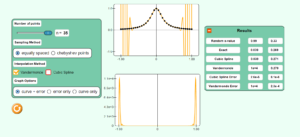# Chapter 05 Higher Order Interpolation is a Bad Idea

Description:  This simulation depicts shows you why conducting higher-order interpolation is a bad idea.  It simulates Runge’s phenomena where he chose several points on a simple smooth function y=1/(1+25x2) in the [-1,1] domain to conduct polynomial interpolation.  We take it a step further to show the use of Chebyshev points and also what happens when a cubic spline is used.Keywords:  Runge’s phenomena, interpolation, higher-order interpolation, cubic spline, Chebyshev points

Learning Objectives:  After successful completion of running the simulation with proper questions asked by the instructor, the student would be able to 1) note the effect of the order of polynomial on the error between the original function and the interpolated polynomial 2) note the effect of the use of Chebyshev points on the higher-order interpolation 3) note how the use of cubic splines improves the representation of the chosen function.

Full Resources:  The full resources for the topic of the higher-order interpolation being a  bad idea, polynomial interpolation,  and spline interpolation include textbook content,  PowerPoint presentations, multiple-choice tests, audiovisual lectures, and application examples.